What Are the Most Popular Data Functions in Excel 2019?

## ?Excel has numerous data functions but understanding a few of the common ones will give you an idea of how any others work.

## The ISERROR Function

If you enter the wrong syntax for a function, forget parameters, or try to perform an invalid calculation, Excel returns an error. For instance, if you attempt to divide by zero in a cell, a "Divide by Zero" error occurs.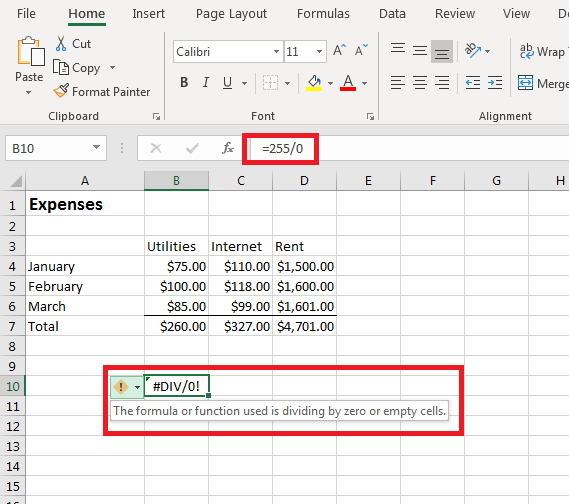(Divide by Zero error displayed in a cell)

There are different types of errors, but any error in your calculations will display with a hash symbol and then the type of error abbreviated. Excel also displays a yellow warning symbol next to the cell with the error. Hover your mouse over the yellow warning symbol, and Excel 2019 displays a short description of the error.

When you unexpectantly have multiple errors across a large range of cells, you might not be able to catch the errors before publishing your content. The ISERROR function will help you find errors throughout your spreadsheets. The ISERROR function takes one parameter, and then it displays "true" or "false" based on the results of the function's evaluation.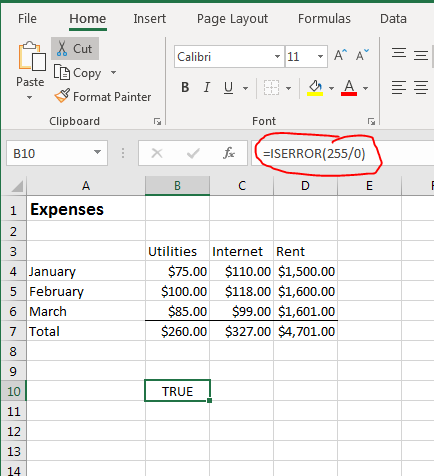(ISERROR function example)

By using your calculations or function calls within an ISERROR statement, you can evaluate and identify any complex calculations return an error. For small spreadsheets, you can review your data and identify errors. With large spreadsheets, you can quickly get a tally on any cells returning an error.

## The ERROR.TYPE Function

Finding errors is useful, but Excel has eight types of errors and it's easier to fix them if you know the type of error. The ERROR.TYPE function will tell you the type of error returned. For instance, if you have nested functions in a division calculation, it could take time to find the function returning a zero with a "divide by zero" error. With the ERROR.TYPE function, you can identify the type of error for a quicker fix.

Some errors are simple typos. For instance, when working with date functions, you can accidentally add a space character in one parameter and return an error. The DATEDIF function requires a third string parameter that tells Excel which part of the date to evaluation. If you add a space to this string value, Excel can't parse the parameter and returns a #NUM! error.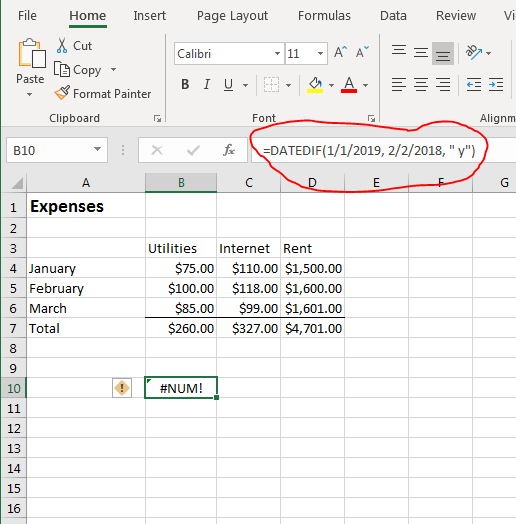(Excel numeric error)

If you skim through the function input, you might miss the space character added to the string, so identifying the error using the ERROR.TYPE function helps fix bugs.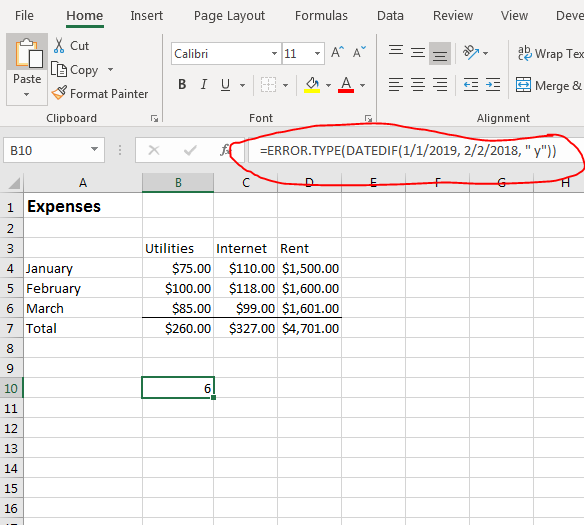(Evaluating an error type)

When you start typing the ERROR.TYPE function parameters, the popup displays the numerical values for each error. When an error is returned, it's displayed as a numerical value. This is useful if you need to find specific types of errors throughout your spreadsheet. Some errors can be ignored, but others cause bugs in your calculations. By having the numeric value displayed, you can add alerts or conditionally format cells such as change the background to red to quickly identify the ones that return a critical type of error.

## The ISNUMBER Function

Some cell data can be numeric or text depending on the type of content that you want to store. You can write formulas and calculations that only add a reference if the cell contains a number. For instance, you might have "N/A" entered into a cell when a value isn't applicable, but then have a numeric value when one is applicable. The ISNUMBER function can identify if a numeric value is stored. It takes one parameter, which is the cell that you want to evaluate.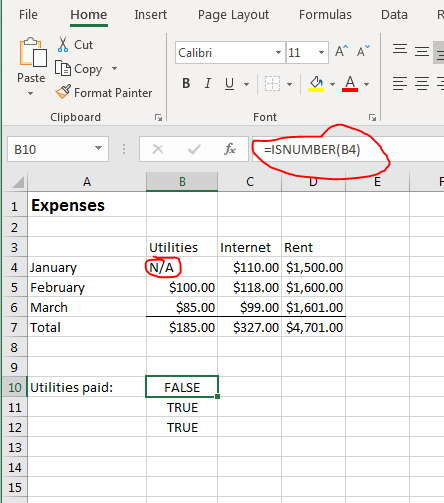(ISNUMBER function example)

Using the "Expenses" example, you might have months that you didn't pay a bill. Using a zero value would indicate that you paid nothing on a bill, but maybe you weren't living in that location at the time, so you have "N/A" entered for these months. Using the ISNUMBER function, you can identify when you have "N/A" versus a value.

Interested in learning more? Why not take an online Advanced Excel 2019 course?

ISNUMBER returns "true" if the referenced cell contains a number. It returns "false" if the value is anything except a value that can be considered a number.

## The ISREF Function

With Excel 2019 formulas, you reference cells frequently in your calculations. The ISREF function will tell you if a cell contains a referenced value (a cell name) rather than a literal value either text or numeric. This function is useful when you need to know that your spreadsheet will be able to dynamically update should any changes be made to your formulas. The ISREF function will also return "false" if a cell contains a calculation that returns a value rather than simply referencing another cell's content.

For instance, the following ISREF function will return "true":

=ISREF(B4:B6)

The above formula returns "true" since the parameter is a reference. If you use references to return a calculated value, the ISREF function will return a "false" value. The following example will return "false":

=ISREF(B4 * B6)

In this example, two cells are used to calculate a result, so the parameter product is a value, not a referenced value. This one distinction between the two results is important when you work with the ISREF function.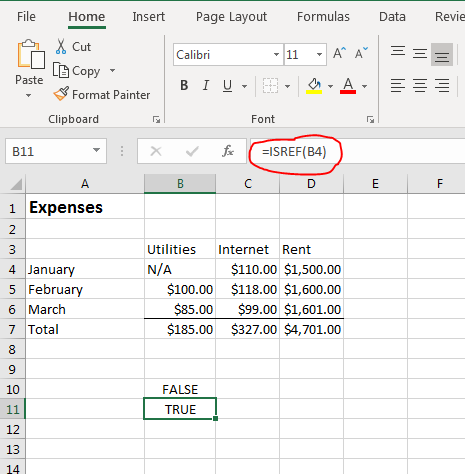(ISREF function example)

In the "Expenses" example, the ISREF function is used to reference B4. Because this is a reference to a cell, the "true" result displays. The "false" cell contains a static value.

## The ISBLANK Function

When you distribute a spreadsheet that requires input, the ISBLANK function displays a "true" or "false" result to let you know if a cell contains a value. When you have hundreds of rows contained in a spreadsheet, this function makes it easier to find blank cells. The ISBLANK function only takes one parameter, which is the cell that you want to evaluate.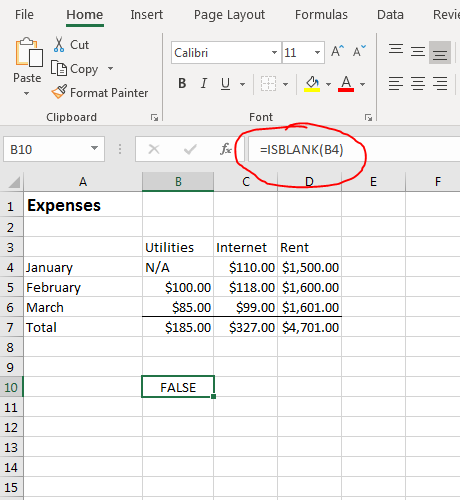(ISBLANK function example)

The parameter for the ISBLANK function is the cell that you want to evaluate. In the example, the B4 contains "N/A" in it, so the function returns false.

## The ISTEXT Function

With Excel forms, it's difficult to control the data entered into cells especially when you distribute it to multiple people to fill out. Excel spreadsheets are also used to collect data from a database or a website. Data is exported to Excel where you can store data either from your own input or from external users. Some functionality such as exporting from Excel into a database requires cells to contain the right data type.

Numeric and textual data are stored differently in databases, and Excel 2019 has functions to detect the data type stored in a cell. The ISTEXT function tells you if a cell contains text and not a formula or numeric value. If the cell contains an error, the ISTEXT also displays "false" in the selected cell. The ISTEXT function only takes one parameter. The parameter is the cell that you want to evaluate.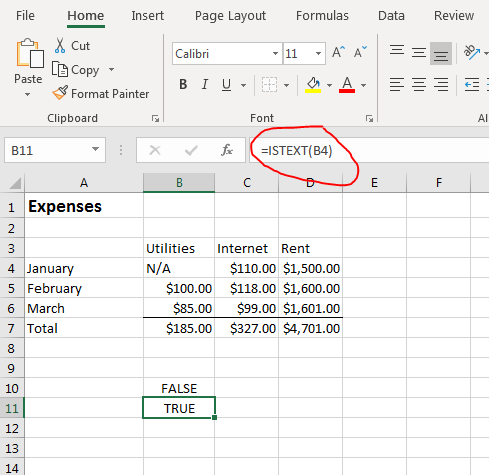(ISTEXT function example)

Just like the other "true" and "false" functions in Excel, the ISTEXT function takes one parameter, evaluates it, and then displays the result. In the "Expenses" example, the B5 cell contains \$100.00, so the result is "false" displayed in the selected cell. The B4 cell contains "N/A" text, so a result of "true" is displayed in the selected cell.

## The ISNONTEXT Function

The ISTEXT function tells you that a cell contains text, but conversely you might want to know if a cell does not contain text. The ISNONTEXT function reverses the evaluation but gives you the same information. With the ISNONTEXT function, you can identify if a cell contains a numeric value, an error, a function or a reference. With this function, you get the opposite response as the ISTEXT function but you can still identify when a cell contains text or some other information.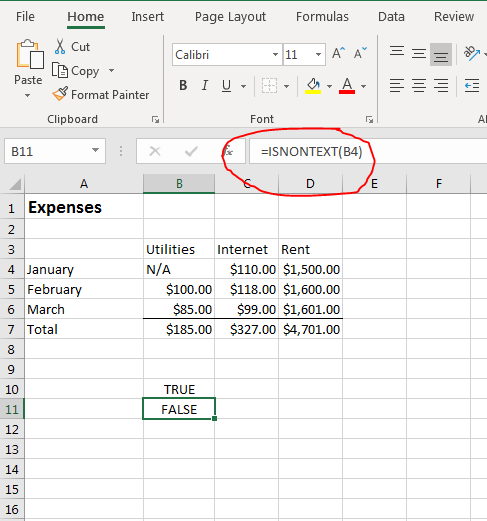(ISNONTEXT function example)

Using the "Expenses" spreadsheet example, changing the ISTEXT function to ISNONTEXT function will return the opposite value. Since the B4 cell contains the "N/A" text value, now the selected cell displays a "false" result. The B5 cell contains the \$100.00 value, so the selected cell displays a "true" result.

## The TYPE Function

Excel 2019 has a few more binary data functions that detect a data type. The ISFORMULA function detects if the cell contains a formula. The ISLOGICAL detects if a cell contains the binary "true" or "false" values. These final two binary functions work the same way as the others mentioned above. They take one cell as a parameter, evaluate the content within the cell, and return a "true" or "false" value. The issue with these functions is that you know if a value matches the condition that it contains a specific data type, but if the cell does not then there are several other data type possibilities. You can detect a more pinpoint data type vale using the Excel TYPE function. The TYPE function returns an integer value that gives you the exact data type contained in the evaluated cell.

The integer values returned are the following:

1 - the cell contains a number

2 - the cell contains text

4 - the cell contains a logical value (true or false)

16 - the cell contains an error value

64 - the cell contains an array of value (e.g. {1,2,3})

With more precise detail about the content in your spreadsheet, you can identify the cells that need updates and the type of content that must be entered.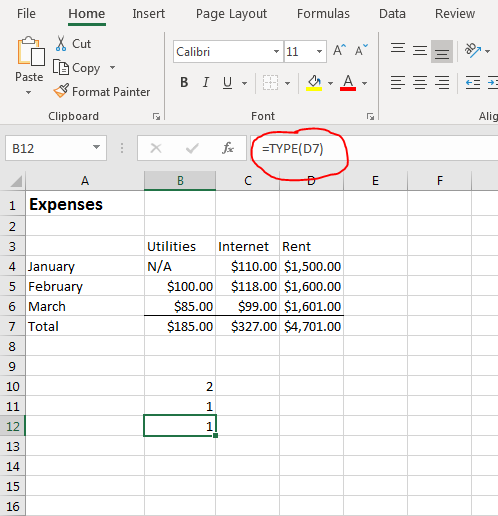(TYPE function example)

Using the "Expenses" spreadsheet as an example, you can see the return values for the cells that contain numbers and those that contain text. The example spreadsheet does not contain logical or error values, but if it did the function would work the same way. The TYPE function evaluates the results of formulas, so you cannot identify if a cell contains formula using this function. Most spreadsheets will contain formulas, and it's mainly important to identify the data type rather than if a cell contains a formula or function.

In the "Expenses" spreadsheet, the "Total" row contains formulas that evaluate to a subtotal numeric value. Because the result is a numeric value, the TYPE function returns a "1" result to the selected cell. This return result tells you that the formula evaluates to a number. The B4 cell contains the "N/A" value, so the result displayed is a "2" value.

Excel has numerous functions, but information functions help you identify the type of data contained within a cell. This can be useful when you have large spreadsheets that require a specific type of data in each cell, but you can't thoroughly review hundreds of rows to ensure that the right information is entered.